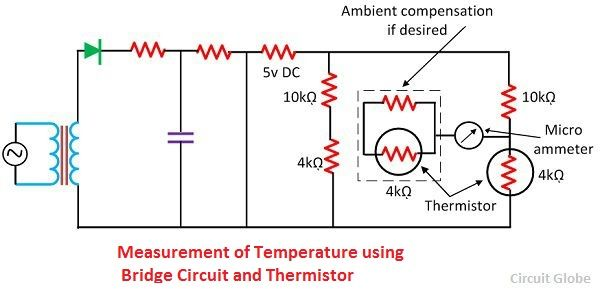# Applications of Thermistors

The thermistor is a thermal resistor whose resistance depends on the external temperature. The measurement and the control of the temperature are the major applications of the thermistor. The thermistor is also used for various other applications which explain below in details.

### 1. Measurement of Temperature

The thermistor is a resistor whose resistance changes with the small change in the measured temperature. The thermistor has good sensitivity, which provides high accuracy and resolution. The industrial thermistor has 2000 ohm resistances at 25º Celsius temperature with 3.9 percent temperature coefficient.Consider a thermistor is connected in series with the ammeter and the battery as shown in the figure below. The small change in temperature causes a change in the resistance of thermistor which relatively changes the current of the circuit. The micrometre is calibrated regarding temperature. The micrometre gives a resolution of about 1ºC.The circuit uses a bridge circuit, and the thermistor shown in the above figure is more sensitive and gives the accurate measurement. The voltage of the standard bridge circuit at 25ºC is approximately equal to the 18mV/ºC, and their circuit uses a 4kΩ thermistor.

### 2. Control of Temperature

The simple temperature circuit is shown in the figure below. The circuit uses a 4kΩ resistor connected in series with the AC excited bridge. The unbalance voltage applied to an amplifier, and the output of the amplifier excites the relay. The relay controls the circuit current and generates heat. These circuits are controlled with an accuracy of 0.00005ºC.The circuits control by the thermistor is stable and sensitive. The response time of the thermistor control circuit is very fast, and their circuits are simple.

### 3. Temperature Compensation

The temperature has a negative temperature coefficient whereas the conductor and the semiconductor have the positive temperature coefficient. Because of this reason the thermistor is used in the circuit for compensating the temperature of both the component and circuit.

The disc type thermistor is used for compensating the temperature not more than 125ºC. The thermistor is mounted near the circuit component like copper meter coil which experiences the same temperature changes of the circuit. The curve below shows the compensation network.The compensator network consists a thermistor connected in parallel with the thermistor. The negative temperature coefficient of the thermistor and the positive temperature coefficient of the circuit component makes the whole circuit temperature constant.

### 4. Other Applications

The thermistor has various other applications.

1. It is used for the measurement of high-frequency power.
2. The thermistor measures the thermal conductivity.
3. The thermistor measures the pressure of the liquid.
4. It measures the composition of gases.
5. The thermistor measures the vacuum and provides the time delays.

The thermistor has more applications as compared to the thermocouple and resistance thermocouples.